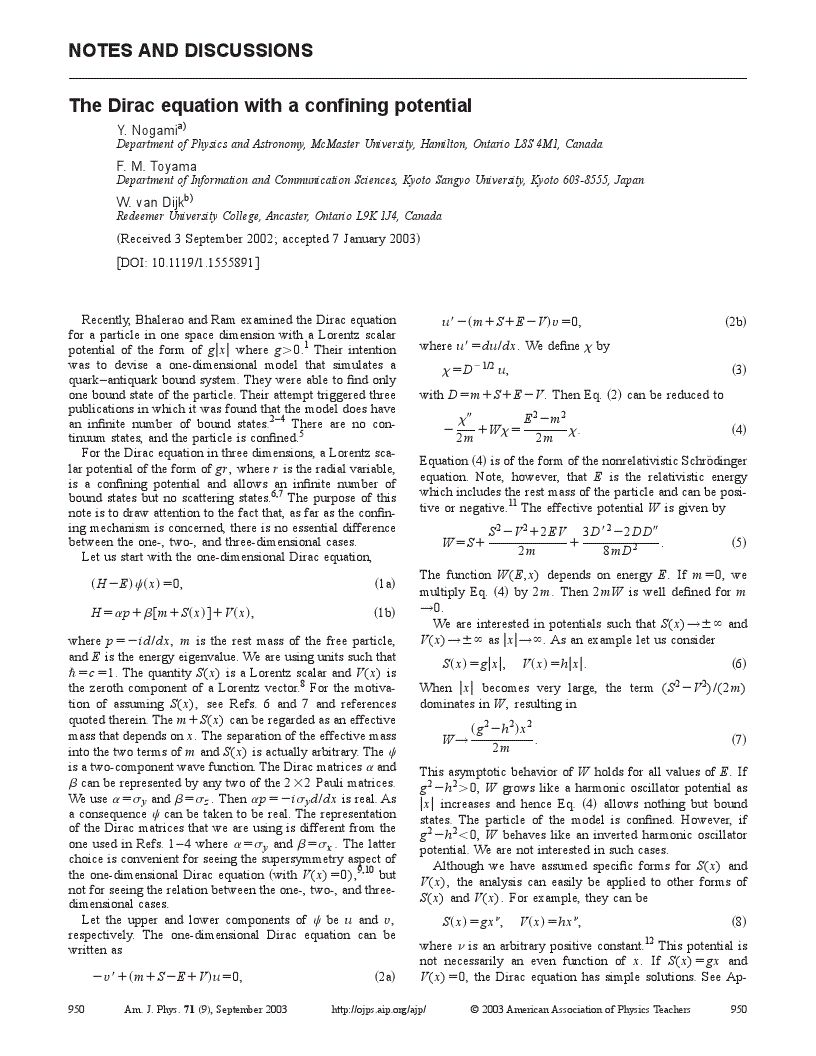### Article PDF first page previewClose Modal
1.
R. S.
Bhalerao
and
Budh
Ram
, “
Fun and frustration with quarkonium in a $1+1$ dimension
,”
Am. J. Phys.
69
,
817
818
(
2001
).
2.
Antonio S.
de Castro
, “
Comment on ‘Fun and frustration with quarkonium in a $1+1$ dimension,’ by R. S. Bhalerao and B. Ram [Am. J. Phys. 69 (7), 817–818 (2001)]
,”
Am. J. Phys.
70
,
450
451
(
2002
).
3.
R. M.
Cavalcanti
, “
Comment on ‘Fun and frustration with quarkonium in a $1+1$ dimension,’ by R. S. Bhalerao and B. Ram [Am. J. Phys. 69 (7), 817–818 (2001)]
,”
Am. J. Phys.
70
,
451
452
(
2002
).
4.
John R.
Hiller
, “
Solution of the one-dimensional Dirac equation with a linear scalar potential
,”
Am. J. Phys.
70
,
522
524
(
2002
).
5.
The solutions of the Dirac equation for the model of Ref. 1 are given in terms of the Hermite function $Hν$ or equivalently, the parabolic cylinder function $Dν.$ The index ν was apparently assumed to be a positive integer in Ref. 1, which is why only one bound state was found. But ν is in general a noninteger as shown in Refs. 2,3,4.
6.
C. L.
Critchfield
, “
Scalar binding of quarks
,”
Phys. Rev. D
12
,
923
925
(
1975
);
C. L.
Critchfield
, “
Scalar potentials in the Dirac equation
,”
J. Math. Phys.
17
,
261
266
(
1976
).
7.
Budh
Ram
, “
Quark confining potential in relativistic equations
,”
Am. J. Phys.
50
,
549
551
(
1982
).
8.
The terminology such as “Lorentz scalar” in the present context is not strictly legitimate. For example, the “scalar potential” $S(x)=g|x|$ is not a Lorentz scalar because $|x|$ is not invariant under the Lorentz transformations. However, we adopt this commonly used terminology.
9.
F.
Cooper
,
A.
Khare
,
R.
Musto
, and
A.
Wipf
, “
Supersymmetry and the Dirac equation
,”
Ann. Phys. (N.Y.)
187
,
1
28
(
1987
).
10.
Y.
Nogami
and
F. M.
Toyama
, “
Supersymmetry aspects of the Dirac equation in one dimension with a Lorentz scalar potential
,”
Phys. Rev. A
47
,
1708
1714
(
1993
).
11.
In Eq. (4) $v$ has been eliminated in favor of χ or $u.$ Alternatively, we can eliminate $u$ in favor of $v$ by redefining χ as $χ=v(m+S−E+V)−1/2.$ This different choice of χ, which we do not use in this note, results in the same physics, that is, the same wave functions (in terms of $u$ and $v)$, and the same energy eigenvalues. In this connection, note that Eq. (2) is invariant under the simultaneous substitutions of $u→v,$$v→u,$$E→−E,$ and $V→−V.$ If $V=0,$ there is a symmetry between positive and negative energy eigenvalues.
12.
If $ν<1$ and if $(g−h)(m+E)<0,$ the last term of $W$ becomes singular and attractive. Then there can be a problem for $W$ in supporting bound states. We exclude such situations. Essentially the same remark applies to the $W(n)$ of Eq. (9).
13.
F. A. B.
Coutinho
and
Y.
Nogami
, “
Zero-range potential for the Dirac equation in two and three space dimensions: Elementary proof of Svenson’s theorem
,”
Phys. Rev. A
42
,
5716
5719
(
1990
).
14.
L. I. Schiff, Quantum Mechanics (McGraw–Hill, New York, 1968), 3rd ed., Chap. 13.
This content is only available via PDF.